# How to Average Every 5 Rows or Columns in Excel?

Sometimes, when we want to calculate the average of every five objects in Excel, we can directly follow a shortcut, which will be mentioned in this article. This will save you a lot of time compared to calculating the average for every five units using the general method. This tutorial will explain how to average every five rows or columns in Excel. We can complete this process by using the formulas.

## Average Every 5 Rows in Excel

Here we will use the AVERAGE and OFFSET formulas to get our first average and use the autofill handle to get all the averages. Let us look at a simple procedure to see how we can average every five rows in Excel.

Step 1

Let us consider an Excel sheet that contains a list of numbers, as shown in the below image.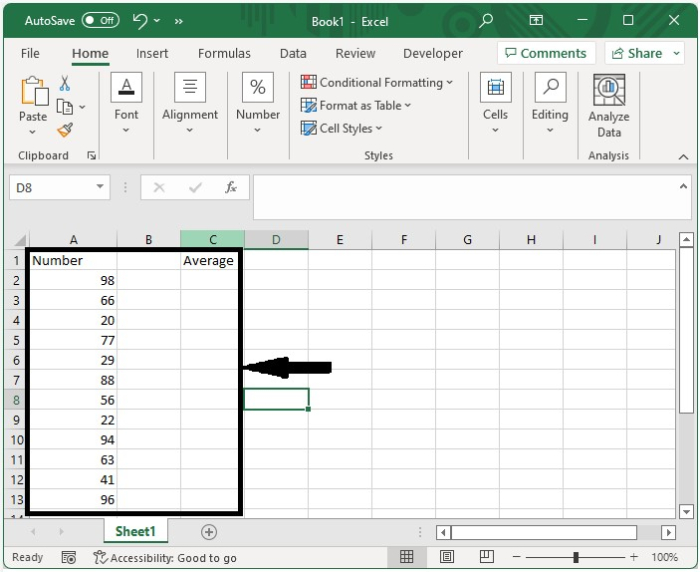Now click on an empty cell and enter the formula as =AVERAGE(OFFSET($A$2,(ROW()-ROW($C$2))*5,,5,)) in the formula box and click OK to get the result for the first five values. In the formula, A2 represents the start value, and C2 is the address where the formula is entered.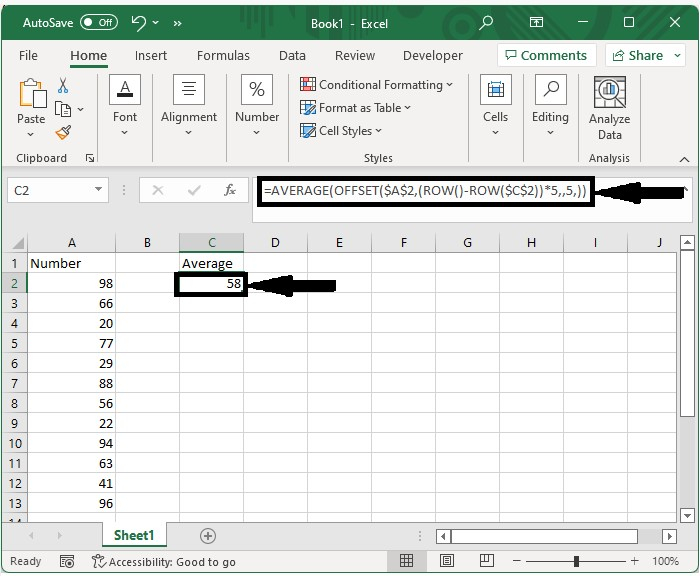Step 2

Now we can get the average of the next five values just by dragging the auto-fill handle. An error will occur if all five values are not available.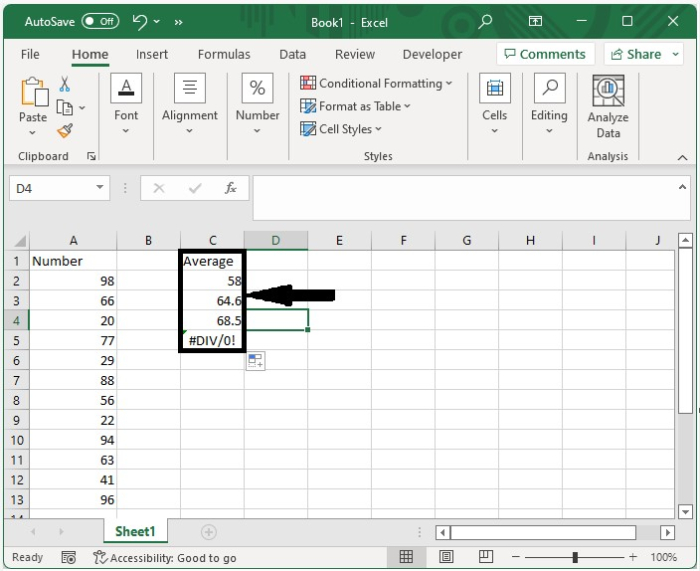## Average Every 5 Columns in Excel

Here we will use the formula to get the result, then use the autofill handle to complete our task. Let us Let us look at an easy method for calculating the average of every five columns in Excel.

Step 1

Let us consider an Excel sheet that contains a list of numbers, as shown in the below image.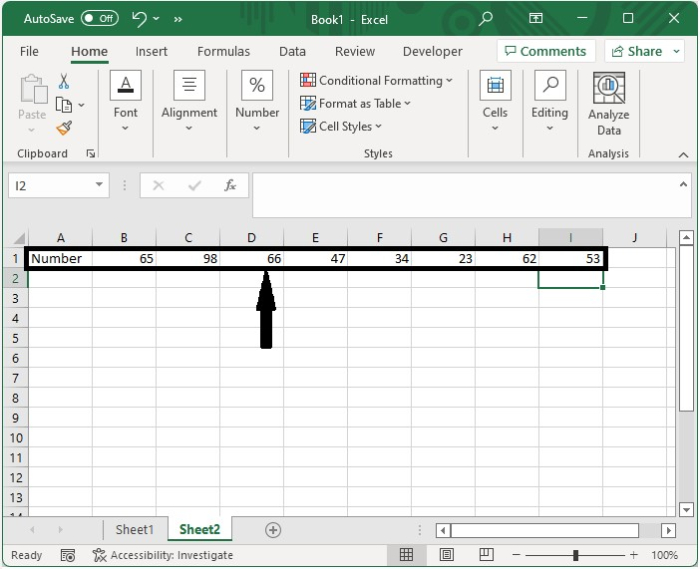Now click on an empty cell and enter the formula as =AVERAGE(OFFSET($A$2,(ROW()-ROW($C$2))*5,,5,)) in the formula box and click OK to get the result for the first five values. In the formula, A2 represents the start value, and C2 is the address where the formula is entered.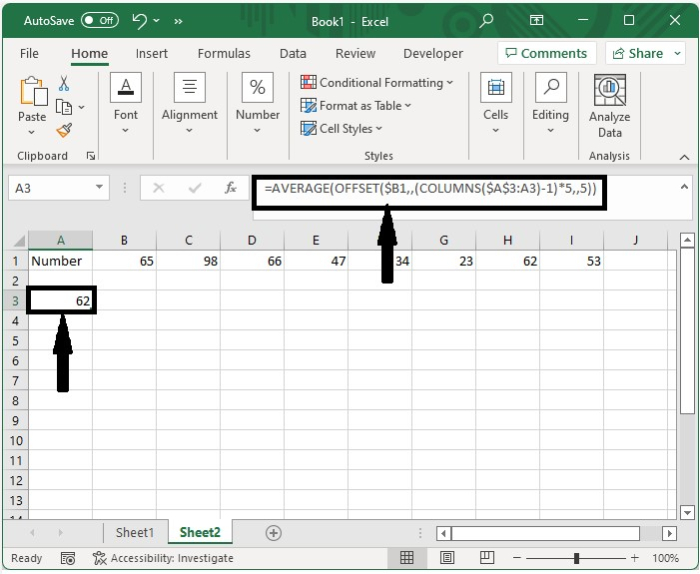Step 2

Now we can get the average of the next five values just by dragging the auto-fill handle. An error will occur if all five values are not available.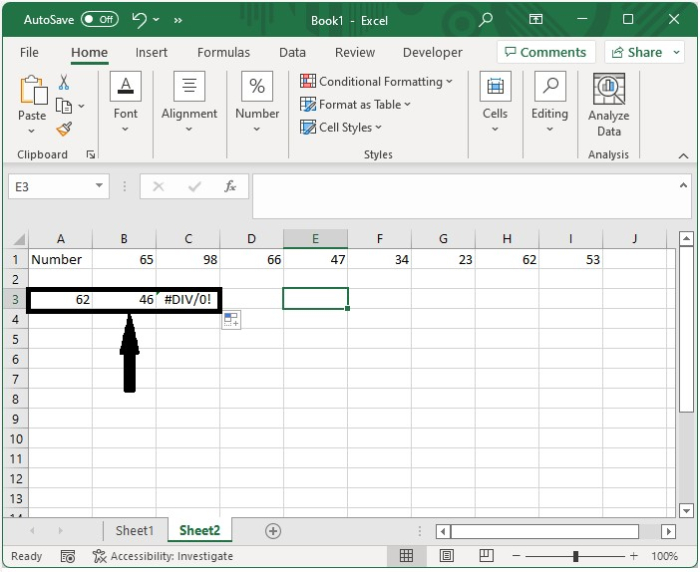## Conclusion

In this tutorial, we used a simple example to demonstrate how we can average every five columns and rows in Excel.

Updated on: 12-Jan-2023

6K+ Views